# How to add & subtract time in Excel to show over 24 hours, 60 minutes, 60 seconds

The article shows a few tips to calculate and display times that are greater than 24 hours, 60 minutes, 60 seconds.

When subtracting or adding time in Excel, you may sometimes want to display the results as the total number of hours, minutes or seconds. The task is a lot easier than it may sound, and you will know the solution in a moment.

## How to display time over 24 hours, 60 minutes, 60 seconds

To show a time interval of more than 24 hours, 60 minutes, or 60 seconds, apply a custom time format where a corresponding time unit code is enclosed in square brackets, like [h], [m], or [s]. The detailed steps follow below:

1. Select the cell(s) you want to format.
2. Right click the selected cells and then click Format Cells, or press Ctrl + 1. This will open the Format Cells dialog box.
3. On the Number tab, under Category, select Custom, and type one of the following time formats in the Type box:
• Over 24 hours: [h]:mm:ss or [h]:mm
• Over 60 minutes: [m]:ss
• Over 60 seconds: [s]

The following screenshot shows the "over 24 hours" custom time format in action: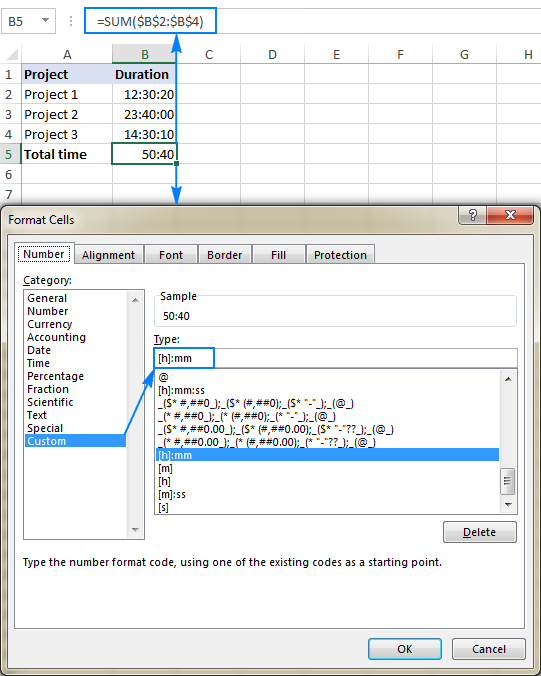Below are a few other custom formats that can be used to display time intervals exceeding the length of the standard time units.

 Description Format code Total hours [h] Hours & minutes [h]:mm Hours, minutes, seconds [h]:mm:ss Total minutes [m] Minutes & seconds [m]:ss Total seconds [s]

Applied to our sample data (Total time 50:40 in the screenshot above), these custom time formats will produce the following results:

A B C
1 Description Displayed time Format
2 Hours 50 [h]
3 Hours & minutes 50:40 [h]:mm
4 Hours, minutes, seconds 50:40:30 [h]:mm:ss
5 Minutes 3040 [m]
6 Minutes & seconds 3040:30 [m]:ss
7 Seconds 182430 [s]

To make the displayed times more meaningful to your users, you can supplement the time unites with the corresponding words, for example:

A B C
1 Description Displayed time Format
2 Hours & minutes 50 hours and 40 minutes [h] "hours and" mm "minutes"
3 Hours, minutes, seconds 50 h. 40 m. 30 s. [h] "h." mm "m." ss "s."
4 Minutes 3040 minutes [m] "minutes"
5 Minutes & seconds 3040 minutes and 30 seconds [m] "minutes and" ss "seconds"
6 Seconds 182430 seconds [s] "seconds"
Note. Although the above times look like text strings, they are still numeric values, since Excel number formats change only the visual representation but not the underlying values. So, you are free to add and subtract the formatted times as usual, reference them in your formulas and use in other calculations.

Now that you know the general technique to display times greater than 24 hours in Excel, let me show you a couple more formulas suited for specific situations.

### Calculate time difference in hours, minutes, or seconds

To calculate the difference between two times in a specific time unit, use one of the following formulas.

#### Time difference in hours

To calculate hours between the start time and end time as a decimal number, use this formula:

(End time - Start time) * 24

To get the number of complete hours, utilize the INT function to round the decimal down to the nearest integer:

`=INT((B2-A2) * 24)`

#### Time difference in minutes

To calculate minutes between two times, subtract the start time from the end time, and then multiply the difference by 1440, which is the number of minutes in one day (24 hours*60 minutes).

(End time - Start time) * 1440

#### Time difference in seconds

To get the number of seconds between two times, multiply the time difference by 86400, which is the number of seconds in one day (24 hours*60 minutes*60 seconds).

(End time - Start time) * 86400

Assuming the start time in A3 and end time in B3, the formulas go as follows:

Hours as a decimal number: `=(B3-A3)*24`

Complete hours: `=INT((B3-A3)*24)`

Minutes: `=(B3-A3)*1440`

Seconds: `=(B3-A3)*86400`

The following screenshot shows the results: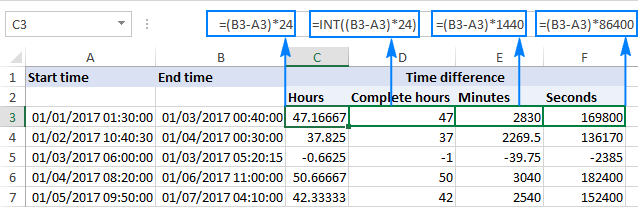Notes:

1. For correct results, the formula cells should be formatted as General.
2. If the end time is greater than the start time, the time difference is displayed as a negative number, like in row 5 in the screenshot above.

## How to add / subtract more than 24 hours, 60 minutes, 60 seconds

To add a desired time interval to a given time, divide the number of hours, minutes, or seconds you want to add by the number of the corresponding unit in a day (24 hours, 1440 minutes, or 86400 seconds), and then add the quotient to the start time.

Start time + (N/24)

Start time + (N/1440)

Start time + (N/86400)

Where N is the number of hours, minutes, or seconds you want to add.

Here're a few real-life formula examples:

To add 45 hours to the start time in cell A2:

`=A2+(45/24)`

To add 100 minutes to the start time in A2:

`=A2+(100/1440)`

To add 200 seconds to the start time in A2:

`=A2+(200/86400)`

Or, you can input the times to add in separate cells and reference those cells in your formulas like shown in the screenshot below: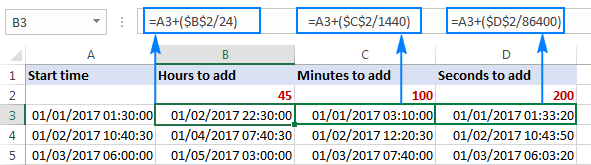#### Subtract over 24 hours:

Start time - (N/24)

#### Subtract over 60 minutes:

Start time - (N/1440)

#### Subtract over 60 seconds:

Start time - (N/86400)

The following screenshot shows the results: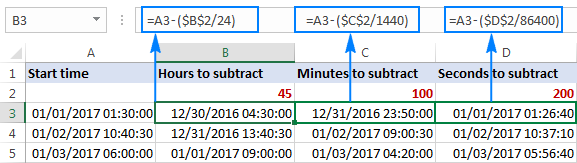Notes:

1. If a calculated time displays as a decimal number, apply a custom date/time format to the formula cells.
2. If after applying custom formatting a cell displays #####, most likely the cell is not wide enough to display the date time value. To fix this, expand the column width either by double-clicking or dragging the right boundary of the column.

This is how you can display, add and subtract lengthy time intervals in Excel. I thank you for reading and hope to see you on our blog next week!

## You may also be interested in

### 70 responses to "How to add & subtract time in Excel to show over 24 hours, 60 minutes, 60 seconds"

1.larry says:

I believe you have an error in the section "How to add / subtract more than 24 hours, 60 minutes, 60 seconds".
"Here're a few real-life formula examples:
To add 45 hours to the start time in cell A2:
=A2+(30/24)"

the formula should be =A2 + (45/24)

•Natalia Sharashova (Ablebits.com Team) says:

Thanks, Larry! Corrected.

•Ino says:

It worked!

2.Imran Masud says:

Thanks, Svetlana.

3.rohan says:

Hi Svetlana, This is very useful and very informative. I have some doubt if have time 12:30 and i apply formula as A2+(2/25) then it decreases the time by 5 Minutes and gives 14:25 time and if apply A2+(2/23) then it increases the time by 5 Minutes and gives 14:35. Could please give me clarity on this how does it calculate?

Thanks, Svetlana again!!

•Svetlana Cheusheva (Ablebits.com Team) says:

Hi Rohan,

I don't know the reasoning behind your calculations, but the results are correct. As you probably know, in internal Excel system, times are stored as decimal fractions, e.g. 00:00:00 is stored as 0.0 and 23:59:59 is stored as 0.99999. So, in terms of times, 2/25 (0.08) is 1:55, when added to 12:30, it gives you 14:25.

4.rohan says:

Thanks Svetlana

5.Indra says:

Hi there!

How should i add running clock in excel?

6.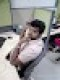Isaiah says:

Thank you so very much. Much appreciated

7.pravin says:

how to without mention date calculation hours check for example 23:00PM -05:30 =06:30

8.MrsH says:

Hi, I need to take a monthly decimal total number of hours worked (i.e. 160.833333) and display as "X hours and Y minutes" which should be 160 hours and 50 minutes, where 49.998 minutes is rounded to the nearest whole minute. I am tearing my hair out.
thanks.

9.v thyagaraj says:

I need to subtract for ex,
10:10 hours from 24 hours,
what formula should i need to use,

Warm regards,
Thyagaraj V

10.Lee Y C says:

suppose I need to add another 30mins to 17.40 hrs. The answer should be 18.10 hrs. Can help me to create a fomula

11.Craig says:

I am compiling a running total of generator running time. I have start and end times in Columns B & C, with format: mm/dd/yy hh:mm. I subtract C from B with formula:

=INT(\$C4-\$B4)&" : "&HOUR(MOD(\$C4-\$B4,1))&" : "&MINUTE(MOD(\$C4-\$B4,1))

Results go into Col. D with results formatted as dd: hh: mm. So far so good, but then I add the individual running times to get cumulative running times in Col. E, and even this works until I get to where the hours exceed 24. They don't add to the days. Example:

2: 23: 43 (d: hh: mm) plus 30 minutes displays as 2: 24: 13 but it should be 3: 0: 13. What do I do to get the correct display?

Thanks,

Craig

12.BB says:

Say my start time is 18:00 hours today and my end time will be 12:00 hours next day. Then how to I calculate the total hours duration (hh:mm format) and also how to get it in decimal format for other calculations (may be y*24).

Many thanks for your kind help.

13.BB says:

I got my answer i.e. 24+(24*(endtime-starttime) = no. of hours difference. I hope I am right in this.

Thanks

14.Khin Lae says:

I'm calculating staff OT for 5PM onward and just wanna show 30minute and above in the cell. Which function can be useful?

If end time is 8:30 PM, i wanna see in the cell is 3:30 as OT and If the end if only 8:15PM. I wanna see in the cell is 3:00 a OT

15.kapil says:

Sir,

16.Natty says:

When I was doing the formula to add how many hours working in hours:minutes, for example 1.17 one day and next day 0.59 total should be showing 2.26 but formula is showing 1.76. How to make a formula if anything over 60 minutes should become a hour?

17.nandu says:

plz solve this problem.
how to calculate 24hours working format....

the labours check in 10-09-2018 5:44AM and they check out next morning 11-09-2018 4:58AM....

BREAK HOUR 2:30Hr
NORMAL WORKING HOUR 08:00Hr....

18.Jolyon Ticer-Wurr says:

Thank you. I figured out how to sum times in Excel, and I've almost figured out how to merge them into a Word document. I say "almost" because Word doesn't seem to be able to handle summed times greater than 24 hours (I'm summing total hours worked).

I've tried every "switch" I can think of, and once the hours:minutes (32:12) goes beyond 24 hours, it will not longer display properly. Is it possible to merge such information to Word and have them display as they display in Excel? (I've not tried using a DDE link because it seems that Microsoft is moving away from this so I didn't want to get attached to something that will be phased out).

19.Josh says:

This isn't working for me. When the total hits 24 hours it starts over; adding time that totals 28:30 shows a total of 4:30.

I am certain both the cells I am adding and the cell I am putting the total in are formatted as custom h:mm.

I am using Excel on an Office 365 subscription; Excel for Mac, version 15.32.

Do you have any further guidance?

•Svetlana Cheusheva (Ablebits.com Team) says:

Hi Josh,

To get an "over 24 hours" custom format, you should enclose the hour code in square brackets. So, the total cell should be formatted [h]:mm

•Yvon says:

Hi Svetlana, can you give an exemple please? thanks

•Yvon says:

Svetlana,
i got it, thanks

20.Ganesh says:

if value of a1 is 2 and value of p1 is 15how to fine vale of b1 c1 d1 e1

21.Trvlbug says:

I am trying to subtract talk time from one year over the next to see if there was a reduction or increase. It works fine if the time decreases but if there is an increase I get the below. 15:01-17:32
give me ######## How do I get it to subtract and give me a negative result

22.goutham says:

if i create 1st date column 2nd, 3rd time column and 4th column again date. if we add 2nd and 3rd column times and get less than 24hrs then 4th column should reflect same date as of 1st column if it is more than 24hrs then 4th column date should be one more tha 1st column. please suggest any formula

23.Becky says:

I searched all over youtube for a formula to show lapsed time from start time and couldn't find the answer. I knew there must be some simple formula, and you had it. Great directions, down to the important details. Many thanks, I took a couple hours trying to find the answer and in five minutes you showed me how. THANK YOU Svetlana for your help. Excel angel!

24.Lemon says:

Hi, i have a questions,
Start date 31/1/2019 10:00:00am and End Date 01/02/2019 11:00:00am
i tried to minus =End date-Start date, but it shows me #value! anyway to solve this?
The cell i need it formula to be [h]:mm:ss

25.TABITHA says:

I am trying to figure out how to add a remainder of time to a total.
Example: time in 8:00 am, time out 5:00 pm = total time 9:00 hours. Allowed 0:30 minute paid break but went over (0:47). How do I add the 0:17 minutes to the total time if allowed time goes over 0,30,0

Thank you

26.Hashan says:

how to calculate total running hours
ex:
last month running Hrs. xxxx (format cell - [H]:mm)
but if I enter more then 4 digit total running Hrs. cell show #VALUE!
Last Month R/HRS 1358:06
TOTAL R/HRS 1358:06
TOTAL ACCUMULATED RUNNING HRS 1358:06 [=SUM(B31+B32)]
But if enter 5 digit show #VALUE!
Last Month R/HRS 13580:06
TOTAL R/HRS 13580:06
TOTAL ACCUMULATED RUNNING HRS #VALUE! [=SUM(B31+B32)]

27.Rajesh Singh says:

Hi,

I have this two time duration in text format(HH:MM:SS). I am trying to add both and find the total time duration of the activity. However the values are not reflecting correctly. It is showing as 0:00 only.
01:40:00
00:05:00
Can anyone please me in understanding the issue.

28.Romel Lumosad says:

Hi,
I am trying to get the difference of the total breaks that my agent is taking. Can anyone tell me here the exact formula in google sheets for this pleaseee 60 minutes minus 00:55:00+00:15:23+00:24:56.

•Natalia Sharashova (Ablebits.com Team) says:

Hi Romel,

if I get it correctly, I believe you can enter these values to your cells (for example, 00:60:00 to A1; 00:55:00 to A2; and so on), apply Duration format to them, and create a simple formula like this: =(A2+A3+A4)-A1
Then set Duration format to the resulting cell as well and you'll see a difference of 00:35:19.

29.Ian Mckay says:

Im really confused because this all seems like it should be easy but I just dont get the correct result.
Example: Start time is 08:40 (A1)
Current time is 10:45 (B1)
Time difference 02:05 (C1)
in D1 I have =C1*1440 but the result is 62,860,445
and I know thats not how many minutes are between 8:40 and 10:45
My Current time cell uses =NOW(), could that be the issue?
Many thanks

30.Sidhant says:

Hi,
I am trying to get a series of value for a column with datetime datatype showing seconds over 60 seconds. For Instance, take the example as "23-06-2019 14:30:59". When I am trying to use the custom format as "dd-mm-yyyy hh:mm:[s]" then it is giving error and throwing some decimal number for "23-06-2019 14:30:60" or "23-06-2019 14:30:61". Can anyone please help me out to get the correct logic for the same
Thanks!

31.David Chapman says:

Hi, I am trying to produce an hourly blood pressure chart in Excel. How do I enter and how do I display the hourly entry of each observation please?

32.Nadya says:

I got it

33.Christopher says:

Hi, i need help please. I have data that auto loads daily at 5pm. The process is broken into 2 parts (ETL1 and ETL2). ETL1 starts at 5pm till 11pm and ETL2 starts at 11 till 4 am. at 7 am i run a script to check if everything ran. each row has a start and end date time. i would like to flag all data after 5pm yesterday as todays data. Currently when i filter on today, i only see the rows where the date is after 00:00

34.Jonathan says:

Hi, I had a similar problem working with time. For 24 hours Excel stores this as 1 day, 0 hours. My solution was to convert everything to seconds and work from there. If you have formatted as days, multiply by 86400 (seconds in a day), if you have formatted as hours, multiply by 3600 (seconds) in an hour, etc.

35.Mary says:

I have a question, If I have 01:40am and i want to minus 20:55:00pm what is the correct formula I need in order for it to see I have gone into the next day? I have tried and it will give me a -19... I need it to see that it is a 24hr period

36.vish says:

this is a time related query.
when i do 23:59 - 23:30, i get an answer as 29 minutes formula=(b1-a1)*1440
when i do 00:15 - 23:59, i get a negative with same formula, this is the problem.
solution required = how do i get the number of minutes? which should be 16 minutes.
Look forward to getting a solution, thanking everyone for their inputs!
:)

37.Bob says:

When I use custom format to represent times greater than 24 hours, the [h]:mm:ss displays 575:00:00.
Why the addition of 551 hours to the total?

•bob says:

Because the [h]:mm actually requires that you convert the total hours to DAYS.fraction. So take (calculated hours)/24. to display with [h]:mm format

38.Swimmer says:

I am trying to show my kid when he improves in his swimming times. It is all good when he improves. Formula works great. But, if he did not improve the formula does not work. For example: last month it took him 00:36.55 (milliseconds) to swim 50 yards. This month it took him 00:35.70. So, he improved 00:00.85.
But, if the numbers are opposite and he did worse the formula will not give me the negative number I am looking for. 00:35.70 - 00:36.85 = -00:00.85
All cells are formatted mm:ss.00;@
Is there a way to show a negative number in time when deducting?

39.Tina Smidt says:

Hi I am trying to create a time sheet that displays elasped time between start time and end time. I have times that cross over 24 hours, where the end time is smaller than the start time. I have been using =mod(end time - start time,1). However this does not work when subtracting a smaller end time from a start time on another date. Is there one formula that I could use for all types of subtraction of time that would also capture the smaller end time - larger start time crossing over 24 hours?

40.swaroop says:

I have 4 machines, which run for few hours in a shift,I note down the number of hours of breakdown/ idle time (In hours) for each machine individually. At the end i want to calculate run time of "all the machines combined" by subtracting the " SUM of idle times of all machines" from 32 hours.

Please suggest a suitable formula/ technique for the same

41.Dixanda says:

Very useful and helpful explanation and information.TQ

42.Melody says:

I need to subtract dates and time where the numerator is MM/DD/YY HH:MM (military time, cell = O8) is in one cell and the denominator is in two separate cells one for date (cell = A8) the other for time (cell = W8).

43.Reda Fouad says:

Thnaks so much

44.Sathish says:

After Computing the formula i got Month 1 Total Extra Hours Worked A1= 17 hours, and computing month 2 total Extra hours Worked A2 = -5 Hours. Now please tell me the formula for getting 17 hrs (-) -5 hrs. How to get 12 hrs as answer.

45.P Maruthi Prasad says:

Thankyou so much for valuable information. It's working obsolutely fine.

46.JERRY says:

HOW CAN I CONVERT 110.5HRS TO DAYS / HOURS / MINUTES IN EXCEL FORMULA

•Alexander Trifuntov (Ablebits.com Team) says:

Hello Jerry!
=A1/24
Then go to Format Cells, choose Number -> Custom Format and set
dd:hh:mm;@
or
dd"d." hh"h."mm"m.";@

47.Adam says:

How do I get 1 hour to show as 60 minutes in excel? Example: it shows 1:00:00.0 currently but would like it to show 60:00.0 instead.

•Alexander Trifuntov (Ablebits.com Team) says:

Please go to Format Cells, choose Number -> Custom Format and set
[mm]:ss.0;@

I hope this will help, otherwise please do not hesitate to contact me anytime.

48.Stan says:

Hi, I'm trying to subtract 2 hours from 01:30:00. It should give me 23:30:00 but I keep getting an "#########" answer.

•Alexander Trifuntov (Ablebits.com Team) says:

Hello Stan!
If the end time is greater than the start time, the following formula should work for you:

=IF(B3 > B1,B1-B3+1,B1-B3)

To the start time you need to add 1 day.

49.Alan says:

Hi,
I have a data dump from a scheduling system that displays the time in excel as follows:
1.00:48:34 - I am reading this as 1 day, 48 minutes, 34 seconds.
1.10:19:09 - 1 day, 10 hours, 19 minutes and 9 seconds.
When I try to add these cells in Excel, I get nothing. What time format can I convert these to and/or what formula could I use?

•Alexander Trifuntov (Ablebits.com Team) says:

Hello Alan!

=LEFT(A1,FIND(".",A1,1)-1)+TIMEVALUE(RIGHT(A1,8))

Please go to Format Cells, choose Number -> Custom Format and set

d"d."h"h."mm"m."ss"s."

I hope this will help, otherwise please do not hesitate to contact me anytime.

50.Maqsood says:

I want to calculate the attendance sheet but the hours total in not coming right.
... .. but when i get the total time it gives wrong answer. i have change formatting using different time format but did not get the right answer.
regard.
Maqsood
time in Time out time in time out
8:30 3:45 8:00 4:00

•Alexander Trifuntov (Ablebits.com Team) says:

Hello!
I’m sorry but your task is not entirely clear to me. For me to be able to help you better, please describe your task in more detail. Please specify what you were trying to find, what formula you used and what problem or error occurred. Give an example of the source data and the expected result.
It’ll help me understand it better and find a solution for you. Thank you.

51.Talha says:

Kindly help this condition
my job time 9:00 am and grace time start 9:15 am
I want to calculate 9:16 am to start late time in minutes

52.Rick says:

Setting up a time card, I have 9 columns, 3 sets of IN time and OUT time. I can get columns to add up using a 24 hour format when it's a low vs a high hour such as IN:0600 / OUT:1600 for a total of 10 hours. However, what I can't figure out is how to formulate In:2000 / OUT:0600 for a total of 10 hours as the amount of time worked was 10 hours.

I know out how to get it to auto sum hours worked when I format the cell (mm/dd/yyyy h:mm) but that over populates the time card and makes it a lot harder to read. Is there a way to auto calculate the total hours worked using (h:mm) and auto sum the 3 column sets into a single total hours worked regardless of what day and time they clocked in?

My crews work 24 hour rotating shifts logging between 8 to 14 hours at a time. They also check out between the start and end of the shift and have to clock back in.

For Example; IN-19:00 (Begin Shift) OUT-24:00 (30 Min Meal) / IN-00-30 OUT-0330 (30 Min Rest) / IN-0400 OUT-0600 (Time to go Home) Total time worked: 10 Hours

I want to set up a time card they can just put in the time they clock in and the time they clock out to simplify their lives. It'll also help keep from displaying unnecessary data for our finance clerk and speed the processing of payroll up.

•Alexander Trifuntov (Ablebits.com Team) says:

Hello Rick!
If the end time is longer than the start time, then you need to add 1 day to their difference

=IF(A1 > A2,A2-A1+1,A2-A1)

If you need the number of hours to be more than 24, use the custom format [h]:mm:ss.
I hope this will help, otherwise please do not hesitate to contact me anytime.

53.Richard says:

Hi excel specialist
How can I change from excel format from 24-00 to 24.00. what is the formula
54.LuTonino says: